Next: Incompressible flow Up: Fluid Mechanics: Preliminaries Previous: Hydrodynamic variables

# The equation of continuity

Having introduced our continuum, or hydrodynamic, variables, we now need to construct equations of motion for these variables. We do this by appealing to conservation laws, such as conservation of mass, momentum, and energy. In this section we will focus on the conservation of mass and derive the equation of continuity for the mass density. Consider an arbitrary, fixed volume V, inside the fluid (see Fig. 2.3).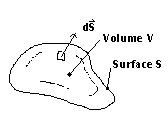Figure 2.3: Fluid volume used for the derivation of the continuity equation.

Fluid is entering and leaving V by crossing the surface S. If we let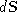be an element of surface area which points outward, normal to the surface, andbe the fluid velocity at the surface, then it is the component ofin the direction ofwhich is responsible for transporting mass out of V. Therefore, the mass flux (mass per unit time) transported through the infinitesimal surface area dS is . Integrating this over the entire surface S, we have the net rate of change of mass in the volume V is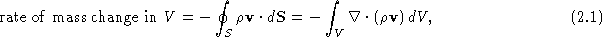where the minus sign has been added since the mass is decreasing, and the last equality follows from the divergence theorem. Now the total mass in the volume V is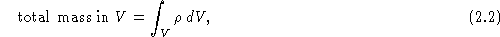and the rate at which this mass is changing with time is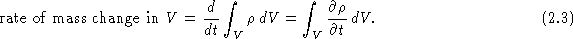Since mass is conserved in an arbitrarily small volume V, the integrands of Eqs. (2.1) and (2.3) must be equal, and we have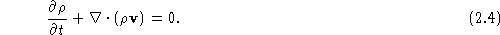This is the equation of continuity, which is a mathematical statement of conservation of mass.

Vittorio Celli
Mon Aug 11 22:46:35 EDT 1997